# 数据集行权限使用案例分享

## 案例一：“一人多店”，用户属性多值匹配

#### 方案：

1. 配置用户属性：在用户属性添加【门店】这一属性，填写用户对应的门店编码；若一人管辖多个门店，则填写多个门店编码，并以分隔符（如逗号）隔开。

2. 行权限配置：在数据集「数据安全」界面设置行权限，条件模式下，添加条件，选择“in(用户属性)”，点选进行界面化配置（此功能5.5及以后版本可用）。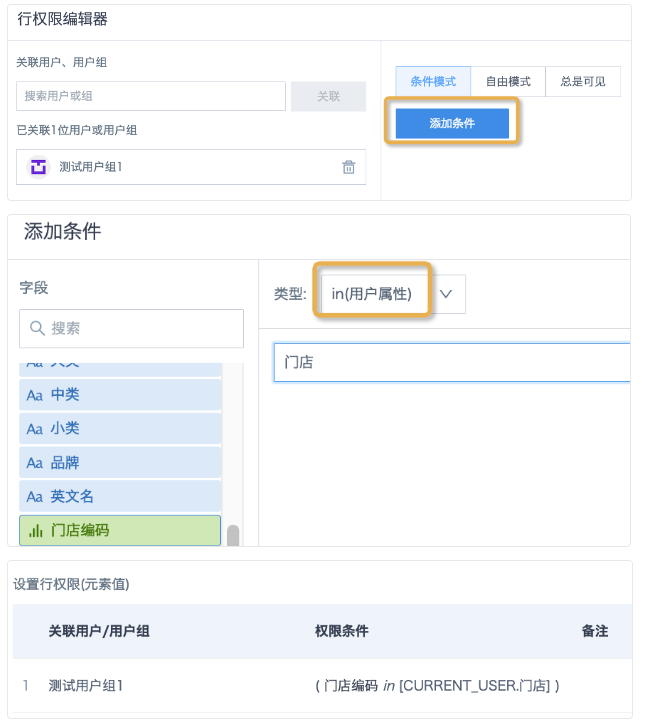3. 5.5以前的版本，可以使用自由模式配置权限条件。

`array_contains(split( [CURRENT_USER.门店] ,','),[门店编码])`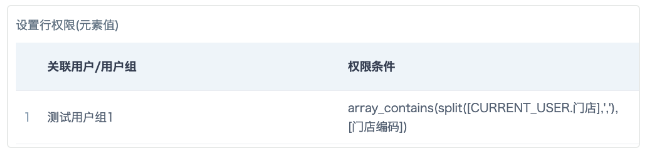Clickhouse写法：

`[门店编码] in splitByChar(',',[CURRENT_USER.门店])`

#### 【注意事项】

1. 用户属性为字符串string格式，数据集字段若非string格式，建议先把字段转换为string格式，再设置行权限；

2. 建议优先使用条件模式下的“in(用户属性)”用法，条件模式无法满足需求时再使用自由模式。

3. 其他数据库语法可以参考：各直连数据库行权限设置语法

## 案例二：用户属性多值+逻辑判断+模糊匹配

非直连（非加速）数据集，字段有大区、省份、城市，大区可以精确匹配，但是省份、城市是全称，例如上海市、杭州市、内蒙古自治区，跟用户属性不能精确匹配。

#### 行权限写法：

`case when [CURRENT_USER.大区]<> '' then array_contains(split([CURRENT_USER.大区],','),[大区]) when [CURRENT_USER.大区]= '' and [CURRENT_USER.城市]<> '' then array_contains(split([CURRENT_USER.城市],','),regexp_replace([城市],'市|地区','')) or array_contains(split([CURRENT_USER.城市],','),replace([省份],'市')) else null end`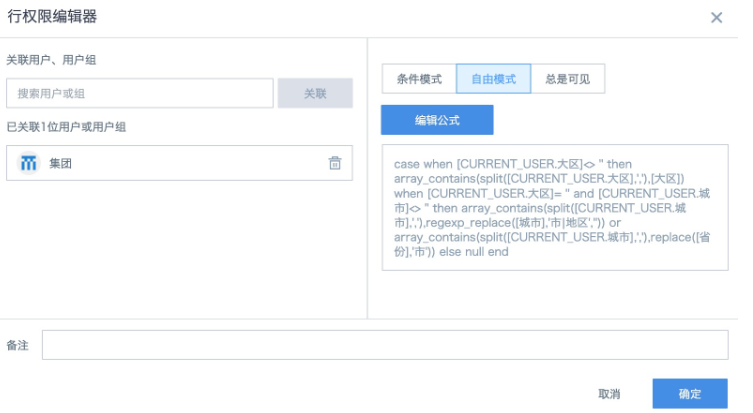#### 逻辑说明：

```-- 判断总公司用户，匹配“大区”字段
case when [CURRENT_USER.大区]<> '' then array_contains(split([CURRENT_USER.大区],','),[大区])
-- 判断子公司用户
when [CURRENT_USER.大区]= '' and [CURRENT_USER.城市]<> ''
-- 把城市字段去掉“市“或者“地区”再进行匹配，如果某些城市名字里带有其他后缀例如”自治州“，可以嵌套使用 replace 函数或者使用正则表达式 regexp_replace来去掉后缀；
then array_contains(split([CURRENT_USER.城市],','),regexp_replace([城市],'市|地区',''))
-- 直辖市城市名如果在省份里，需要匹配"省份"
or array_contains(split([CURRENT_USER.城市],','),replace([省份],’市’))```

#### 【注意事项】：

1. 如果用户属性是字符串单值，可以直接使用 [CURRENT_USER.大区]=[大区] 这样的精确匹配，和 instr([城市],[CURRENT_USER.城市])>0 这样的模糊匹配。

2. 多值的情况下要用 array_contains(split()) 函数逐个匹配（同时也完全适应单值的情况）； 不过数组不适用模糊匹配，不能用 like, 所以需要对数据集字段做处理。位置和长度都一样的情况可以使用 substr 来代替 replace，例如 array_contains(split([CURRENT_USER.城市],','),substr([城市],1,2)) 。

#### Clickhouse写法：

`case when [CURRENT_USER.大区]<> '' then has(splitByChar(',',[CURRENT_USER.大区]),[大区]) when [CURRENT_USER.大区]='' and [CURRENT_USER.城市]<>''then has(splitByChar(',',[CURRENT_USER.城市]),replaceRegexpOne([城市],'市|地区','')) or has(splitByChar(',',[CURRENT_USER.城市]),replaceOne([省份],'市','')) else null end`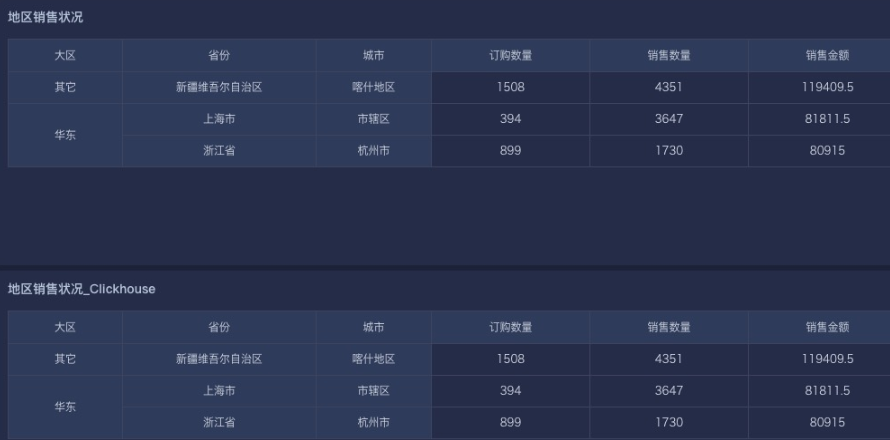## 案例三：用户属性多值+只匹配第一个值+模糊匹配

案例一同一数据集，非直连（非加速）数据集，省份、城市是全称，例如上海市、杭州市、内蒙古自治区，跟用户属性不能精确匹配。普通城市名需要去掉“市”“地区”之类后缀，直辖市需要去掉“市”再跟省份匹配。

#### 行权限写法一：

`array_position(split([CURRENT_USER.城市],','),regexp_replace([城市],'市|地区',''))=1 or array_position(split([CURRENT_USER.城市],','),replace([省份],'市'))=1`

1. 用 split([CURRENT_USER.城市],',') 把城市拆分成数组。

2. array_position()=1 确保提取的是数组中第一个元素，即使用户属性是单值也适用。

#### 行权限写法二：

```case when INSTR([CURRENT_USER.城市],',')>1 then SUBSTR([CURRENT_USER.城市],0,INSTR([CURRENT_USER.城市],',')-1) in (regexp_replace([城市],'市|地区',''),replace([省份],'市'))
else [CURRENT_USER.城市] in (regexp_replace([城市],'市|地区',''),replace([省份],'市')) end```

1. 把用户属性“城市”按照字符串处理。

2. 用 case when 判断是单值还是多值，多值时截取第一个分隔符前的城市名，单值直接匹配城市/省份。

#### Clickhouse写法

`arrayElement(splitByChar(',',[CURRENT_USER.城市]),1) = replaceOne([省份],'市','') or arrayElement(splitByChar(',',[CURRENT_USER.城市]),1) =replaceRegexpOne([城市],'市|地区','')`
```case when position([CURRENT_USER.城市],',')>1 then substring([CURRENT_USER.城市],1,position([CURRENT_USER.城市],',')-1) = replaceRegexpOne([城市],'市|地区','') or substring([CURRENT_USER.城市],1,position([CURRENT_USER.城市],',')-1) = replaceOne([省份],'市','')
else [CURRENT_USER.城市]= replaceRegexpOne([城市],'市|地区','') or [CURRENT_USER.城市]= replaceOne([省份],'市','') end```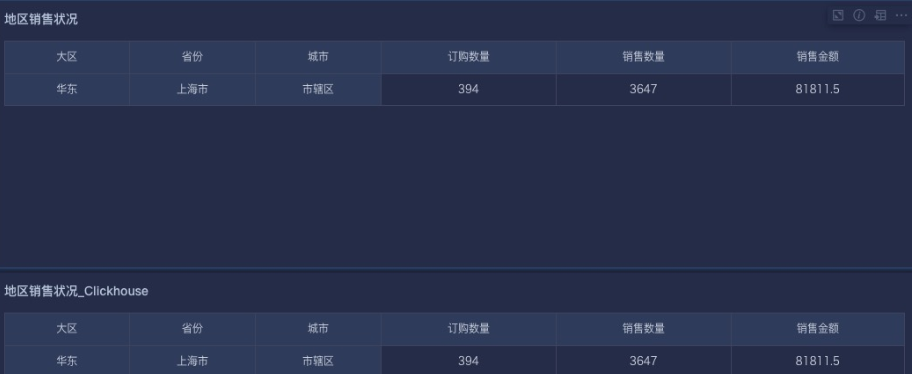## 案例四：仅允许特定用户查看180天内的数据

#### 行权限写法：

`(current_date()-INTERVAL 180 day) <= [日期]`

#### 逻辑说明：

1. 使用current_date() 函数获取当前日期，通过 INTERVAL 可以进行增减日期时间，所以这里通过(current_date()-INTERVAL 180 day) 或者(current_date()-INTERVAL 6 month)  可以取到当前日期-180天（或6个月）的具体日期，再小于等于数据集中的日期数据，即可将看到的数据控制在180天内。

2. 多个规则需要同时生效时，用 and/or 连接即可。例如：

`array_contains(split([CURRENT_USER.属性A] ,','),[分组]) and (current_date()-INTERVAL 180 day)<=[日期]`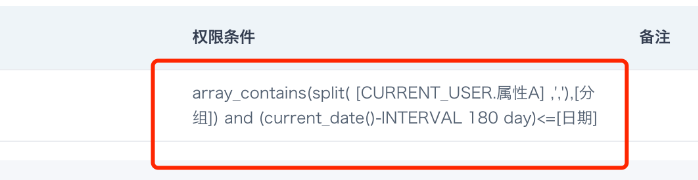9 人点赞过